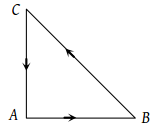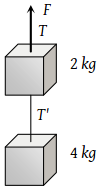A ball of mass 150g starts moving with an acceleration of 20 m/s2. When hit by a force, which acts on it for 0.1 sec. The impulsive force is

(1) 0.5 N-s

(2) 0.1 N-s

(3) 0.3 N-s

(4) 1.2 N-s

Concept Questions :-

Newton laws
High Yielding Test Series + Question Bank - NEET 2020

Difficulty Level:

A body, whose momentum is constant, must have constant

(1) Force

(2) Velocity

(3) Acceleration

(4) All of these

Concept Questions :-

Newton laws
High Yielding Test Series + Question Bank - NEET 2020

Difficulty Level:

Rocket engines lift a rocket from the earth surface because hot gas with high velocity

(1) Push against the earth

(2) Push against the air

(3) React against the rocket and push it up

(4) Heat up the air which lifts the rocket

Concept Questions :-

Variable mass system
High Yielding Test Series + Question Bank - NEET 2020

Difficulty Level:

A force of 50 dynes is acted on a body of mass 5 g which is at rest for an interval of 3 seconds, then impulse is

(1) $0.15×{10}^{-3}\text{\hspace{0.17em}}Ns$

(2) $0.98×{10}^{-3}\text{\hspace{0.17em}}Ns$

(3) $1.5×{10}^{-3}\text{\hspace{0.17em}}Ns$

(4) $2.5×{10}^{-3}\text{\hspace{0.17em}}Ns$

Concept Questions :-

Newton laws
High Yielding Test Series + Question Bank - NEET 2020

Difficulty Level:

A bullet of mass 0.1 kg is fired with a speed of 100 m/sec, the mass of gun is 50 kg. The velocity of recoil is

1. 0.2 m/sec

2. 0.1 m/sec

3. 0.5 m/sec

4. 0.05 m/sec

Concept Questions :-

Newton laws
High Yielding Test Series + Question Bank - NEET 2020

Difficulty Level:

Two forces of magnitude F have a resultant of the same magnitude F. The angle between the two forces is

(1) 45°

(2) 120°

(3) 150°

(4) 60°

Concept Questions :-

Newton laws
High Yielding Test Series + Question Bank - NEET 2020

Difficulty Level:

Two forces with equal magnitudes F act on a body and the magnitude of the resultant force is F/3. The angle between the two forces is

(1) ${\mathrm{cos}}^{-1}\left(-\frac{17}{18}\right)$

(2) ${\mathrm{cos}}^{-1}\left(-\frac{1}{3}\right)$

(3) ${\mathrm{cos}}^{-1}\left(\frac{2}{3}\right)$

(4) ${\mathrm{cos}}^{-1}\left(\frac{8}{9}\right)$

Concept Questions :-

Newton laws
High Yielding Test Series + Question Bank - NEET 2020

Difficulty Level:

Three forces starts acting simultaneously on a particle moving with velocity $\stackrel{\to }{v}.$ These forces are represented in magnitude and direction by the three sides of a triangle ABC (as shown). The particle will now move with velocity(1) $\stackrel{\to }{v}$ remaining unchanged

(2) Less than $\stackrel{\to }{v}$

(3) Greater than $\stackrel{\to }{v}$

(4) $\stackrel{\to }{v}$ in the direction of the largest force BC

Concept Questions :-

Application of laws
High Yielding Test Series + Question Bank - NEET 2020

Difficulty Level:

Two blocks are connected by a string as shown in the diagram. The upper block is hung by another string. A force F applied on the upper string produces an acceleration of 2 m/s2 in the upward direction in both the blocks. If T and T’ be the tensions in the two parts of the string, then(1) T = 70.8 N and T’ = 47.2 N

(2) T = 58.8 N and T’ = 47.2 N

(3) T = 70.8 N and T’ = 58.8 N

(4) T = 70.8 N and T’ = 40.2 N

Concept Questions :-

Application of laws
High Yielding Test Series + Question Bank - NEET 2020

Difficulty Level:

If two forces of 5 N each are acting along X and Y axes, then the magnitude and direction of resultant is

(1) $5\sqrt{2},\text{\hspace{0.17em}\hspace{0.17em}}\pi /3$

(2) $5\sqrt{2},\text{\hspace{0.17em}\hspace{0.17em}}\pi /4$

(3) $-5\sqrt{2},\text{\hspace{0.17em}\hspace{0.17em}}\pi /3$

(4) $-5\sqrt{2},\text{\hspace{0.17em}\hspace{0.17em}}\pi /4$

Concept Questions :-

Types of forces